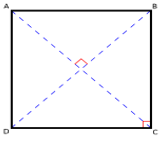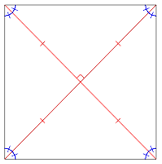Square Formula

Square Formula - Equations & Solved Examples

A square is a unique four-sided polygon which is equilateral as well as equiangular. All the four angles of a square are right angles, each equal to 360°/4, which is equal to 90°.
In this section, we are going to learn about all the formulae related to square which will help us in computing its area, perimeter and the lengths of its diagonals.Properties of a Square
• The diagonals of a square bisect each other and meet at 90°

• The diagonals of a square bisect its angles.

• Opposite sides of a square are both parallel and equal in length.

• All four angles of a square are equal.

• All four sides of a square are equal.

• The diagonals of a square are equal

•Formulas
• 1. Perimeter

• The perimeter of a square having length of four sides as ‘a’ is
Perimeter = 4a.
• 2. Length of a Diagonal of a Square

• It can be easily computed using Pythagoras theorem,
Diagonal = 2a
Area = a2 = (3)2 = 9 cm2